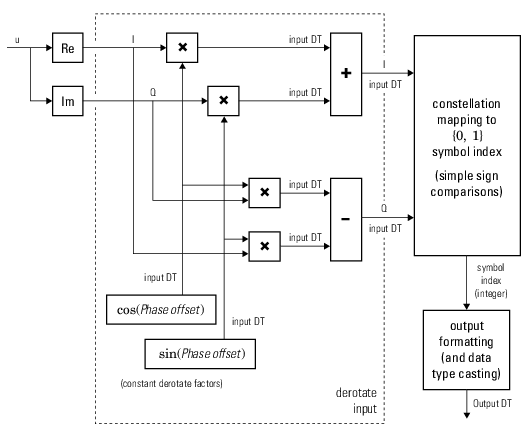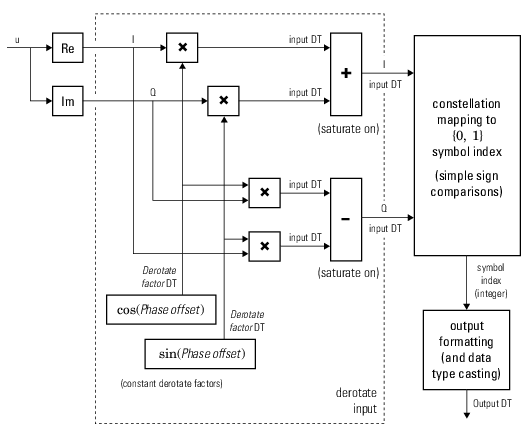Documentation

BPSK Demodulator Baseband

Demodulate BPSK-modulated data

Library

PM, in Digital Baseband sublibrary of ModulationDescription

The BPSK Demodulator Baseband block demodulates a signal that was modulated using the binary phase shift keying method. The input is a baseband representation of the modulated signal. This block accepts a scalar or column vector input signal. The input signal must be a discrete-time complex signal. The block maps the points exp(jθ) and -exp(jθ) to 0 and 1, respectively, where θ is the Phase offset parameter.

For information about the data types each block port supports, see Supported Data Types.

Algorithm

Hard-Decision BPSK Demodulator Signal Diagram for Trivial Phase Offset (multiple of π/2)Hard-Decision BPSK Demodulator Floating-Point Signal Diagram for Nontrivial Phase OffsetHard-Decision BPSK Demodulator Fixed-Point Signal Diagram for Nontrivial Phase OffsetThe exact LLR and approximate LLR cases (soft-decision) are described in Exact LLR Algorithm and Approximate LLR Algorithm in the Communications Toolbox™ User's Guide.

Dialog BoxThe phase of the zeroth point of the signal constellation.

Decision type

Specifies the use of hard decision, LLR, or approximate LLR during demodulation. The output values for Log-likelihood ratio and Approximate log-likelihood ratio are of the same data type as the input values. See Exact LLR Algorithm and Approximate LLR Algorithm in the Communications Toolbox User's Guide for algorithm details.

Noise variance source

This field appears when Approximate log-likelihood ratio or Log-likelihood ratio is selected for Decision type.

When set to Dialog, the noise variance can be specified in the Noise variance field. When set to Port, a port appears on the block through which the noise variance can be input.

Noise variance

This parameter appears when the Noise variance source is set to Dialog and specifies the noise variance in the input signal. This parameter is tunable in normal mode, Accelerator mode and Rapid Accelerator mode.

If you use the Simulink® Coder™ rapid simulation (RSIM) target to build an RSIM executable, then you can tune the parameter without recompiling the model. This is useful for Monte Carlo simulations in which you run the simulation multiple times (perhaps on multiple computers) with different amounts of noise.

The LLR algorithm involves computing exponentials of very large or very small numbers using finite precision arithmetic and would yield:

• Inf to -Inf if Noise variance is very high

• NaN if Noise variance and signal power are both very small

In such cases, use approximate LLR, as its algorithm does not involve computing exponentials.

Data Types Pane for Hard-DecisionOutput

When Decision type is set to Hard decision, the output data type can be set to 'Inherit via internal rule', 'Smallest unsigned integer', double, single, int8, uint8, int16, uint16, int32, uint32, or boolean.

When this parameter is set to 'Inherit via internal rule' (default setting), the block will inherit the output data type from the input port. The output data type will be the same as the input data type if the input is a floating-point type (single or double). If the input data type is fixed-point, the output data type will work as if this parameter is set to 'Smallest unsigned integer'.

When this parameter is set to 'Smallest unsigned integer', the output data type is selected based on the settings used in the Hardware Implementation pane of the Configuration Parameters dialog box of the model. If ASIC/FPGA is selected in the Hardware Implementation pane, the output data type is the ideal minimum one-bit size, i.e., ufix(1). For all other selections, it is an unsigned integer with the smallest available word length large enough to fit one bit, usually corresponding to the size of a char (e.g., uint8).

Derotate factor

This parameter only applies when the input is fixed-point and Phase offset is not a multiple of π/2.

This can be set to Same word length as input or Specify word length, in which case a field is enabled for user input.

Data Types Pane for Soft-DecisionWhen Decision type is set to Log-likelihood ratio or Approximate log-likelihood ratio, the output data type is inherited from the input (e.g., if the input is of data type double, the output is also of data type double).

Supported Data Types

PortSupported Data Types

Input

• Double-precision floating point

• Single-precision floating point

• Signed fixed point (only for Hard decision mode)

Var

• Double-precision floating point

• Single-precision floating point

Output

• Double-precision floating point

• Single-precision floating point

• Boolean

• 8-, 16-, and 32-bit signed integers

• 8-, 16-, and 32-bit unsigned integers

• ufix(1) in ASIC/FPGA and when Decision type is Hard decision modes

Pair Block

BPSK Modulator Baseband